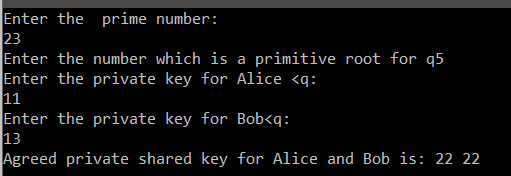What is Diffie Hellman?
It is an asymmetric key exchange algorithm which allows two parties to agree upon a shared secret key in such a way that no other person can eavesdrop on the communication. It uses two different keys for the exchanging purpose i.e, Public key and Private key. It is not an encrypting algorithm but it is used to share the keys that we will further use for the encryption.

In Diffie Hellman, we don’t exactly exchange the keys,but what we exchange are the variables and then both the communicating parties agree on a shared secret key which will then help conduct symmetric encryption of the messages throughout the communication. It is the simplest and commonly used Key exchange algorithm which is impossible to guess because
1. It is computed using exponential Modulo.
2. Both parties uses their own private key for the exchange.
Because of these two reasons the number of guesses are so many that it is impossible to carry out in a single life span.Let’s have a look the steps involved in Diffie Hellman:
Note: A and B are the two parties exchanging the keys
1. Public global generator variables
a. q – prime number
b. a – Should be less than q and a primitive root of q
2. Private key generation for A where private key variable XA < q and with this A create a public key

PubA = aXA mod q
3. B will create its own private key, XB where XB < q and with this B create its public key

PubB =aXB mod q
4. The public keys in both 2 and 3 steps will then be exchanged and computed exponentially with their respective private keys again to form a shared secret Key.
5. For A this Shared secret Key K will be
i. K = (PubB) XA mod q //Here we use exchanged key of B,PubB to compute with private key of A.
6. For B, this shared key K will be
i. K = (PubA) XB mod q //Here we use exchanged key of A,PubA to compute with private key of B.

**NOTE: Here the number q matters, the larger the q the harder it would be for the adversary to crack the code, basically impossible. and also it is impossible to guess the private keys for both the parties. Even if the number q and a are known still the adversary could not be able to find the actual keys because it is mathematically not impossible to evaluate the key with the given public variables and hence it is the most
safest approach yet. But it is still susceptible to Man in the Middle attack where in the adversary is sitting between the channel the communication parties and is able to intercept their messages.
Here is Pictorial representation of Diffie Hellman Algorithm:The K variable above is the shared private key that both Alice and Bob are agreed upon for sharing their messages and If you look at the values of both the K above, It will always give out the same key for both the parties.

C Code For Diffie Hellman:
``` #include<stdio.h> #include<math.h> main(){ unsigned long long int q,a,puba,pubb,privA,privB,ka,kb; printf("Enter the prime number:\n"); scanf("%llu",&q); printf("Enter the number which is a primitive root for q"); scanf("\n%llu",&a); printf("Enter the private key for Alice <q:\n");scanf("%llu",&privA); printf("Enter the private key for Bob<q:\n");scanf("%llu",&privB); puba=((long long int )pow(a,privA)); puba=(long long int)puba%q; pubb=((long long int)pow(a,privB)); pubb=pubb%q; ka=((long long int)pow(pubb,privA))%q; kb=((long long int)pow(puba,privB))%q; printf("Agreed private shared key for Alice and Bob is: %llu %llu",ka,kb); return 0; } ```
OUTPUT:This site uses Akismet to reduce spam. Learn how your comment data is processed.